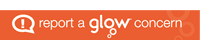# H Computing Science Working From Home

Monday 1st February 2021

This week, there is only one assignment. This assignment is a mix of all Floating Point Binary exercises so far. To help with this, I have created a Floating Point Representation PowerPoint which will take you through step-by-step how to answer each of the different types of questions that can be asked.

The assignment is here: Floating Point Binary Recap

The deadline for this assignment is Friday 3pm.

Monday 25th January 2021

This week, there will be another three assignments for you to complete:

Like last week, the Floating Point exercises will follow the same format with 2 worked examples and a table to complete. In exercise 2, the examples will involve moving the decimal point to the right instead of left. In exercise 3, the examples will involve using negative numbers. Exercise 4 will combine both type of examples from exercises 2 and 3. Please read through the definitions and worked examples carefully before attempting the questions.

The deadline for these three assignments is Friday 3pm.

Monday 18th January 2021

This week, there will be another three assignments for you to complete:

To help you with the Two’s Complement Recap, I have created a PowerPoint that will take you through each of the examples step-by-step. Two’s Complement Recap I was very impressed with your answers last week, but there were a couple of minor mistakes that will just take practice to overcome.

In the Floating Point exercises, we are going to be making use of scientific notation which you might recognise from Maths. We will also be going into more detail about the mantissa and exponent which you learnt about at National 5 level. Reminder, the mantissa stores the precision of the number, and the exponent stores the range of the number. Please read through the definitions and worked examples carefully before attempting the questions.

The deadline for these three assignments is Friday 3pm.

Monday 11th January 2021

The final topic of the Higher Computing Science course is Computer Systems. The first thing we will go over is how to convert numbers from denary (numbers such as 57, 3, 1001 etc.) into binary (numbers that consist of only 1s and 0s).

In task 1, you will see worked examples that show how numbers can be converted into and from binary. These worked examples will also include detailed step-by-step descriptions. You will then have a list of questions to complete.

In task 2, you will move on to negative numbers. The main difference with this exercise is that the first bit used (normally 128) is now changed to represent whether a number is negative or not. Again, there are worked examples with detailed descriptions to help you before you attempt the questions.

In task 3, you will be given a formula that can be used to determine the range of numbers that can be represented using a certain number of bits. You will need to use this formula to find the answers for the number of bits provided in each question.

Complete the revision exercise found here: N5 Binary Revision

Complete the negative numbers exercise found here: H Negative Binary